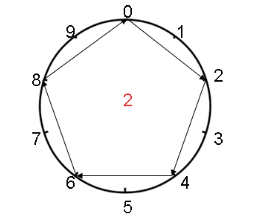You've reached the end of your complimentary access. Subscribe for as little as \$4/month.

Subscribe

Did you know math can be art? I have been doing virtual school since March and I came across in one of my assignments a way of drawing a times table. First, you need to draw a circle like a clock. Make 0 be the 12 on the clock and make 5 be across from 0. It should look like this:Let’s assume you want to draw the 2 times tables. To do that, you need to draw a line from 0 to 2, 2 to 4 {2 x 2 = 4}, 4 to 6 {2 x 3 = 6}, 6 to 8, and back to 0 because 2 x 5 = 10 and the last digit ends with 0. Your picture should look like this:Things start to look more interesting when you do the 3 times table. Always start at 0. Draw a line from 0 to 3, 3 to 6 {3 x 2 = 6}, 6 to 9, 9 to 2 because 3 x 4 = 12 and the last digit is 2, 2 to 5, 5 to 8, 8 to 1, 1 to 4, 4 to 7, and 7 back to 0. Your picture should end up looking like this:You will find that the shape, a pentagon, is exactly like the shape in the 2 times table. There is one difference though. In the 2 times table, you are going clockwise to make the pattern, but in the 8 times table, you are going counterclockwise.

Try other multiplication tables like 4, 6, etc., and see what cool shapes you find! Who knew art and math blend so well together?!